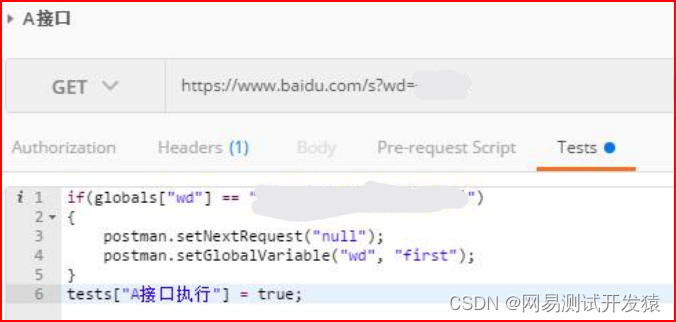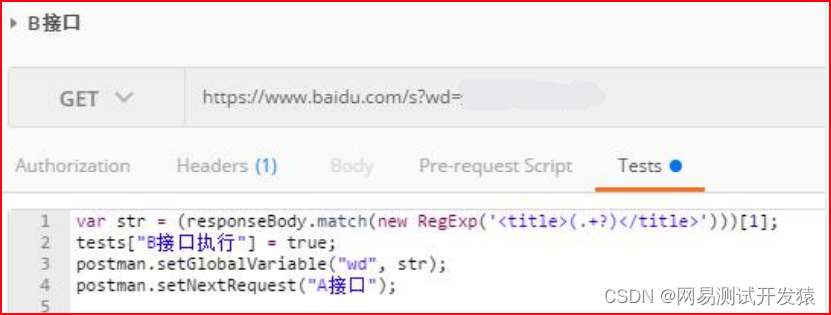0

# 全网最强最细postman接口测试教程（三）

## 一、提取接口返回值

``````var Content-Type = postman.getResponseHeader("Content-Type");
``````

``````var xsrfCookie = postman.getResponseCookie("csrf_token");
``````

3、获取响应的正文

``````var response = responseBody;
``````

4、使用正则表达式截取响应部分内容，通过要截取的字符串左右边界截取

``````var str =(responseBody.match(newRegExp('"expires_in":(.+?)}')));
``````

5、返回的是json数据，可以通过转换成json对象然后解析

``````var responseDate =JSON.parse(responseBody);
responseDate.expires_in
``````

6、转化XML格式的响应成JSON对象—Convert XML body to a JSONobject

``````var jsonObject =xml2Json(responseBody);
``````

## 二、接口关联（串行传参）

1、利用postman获取上一个接口指定的返回值

2、使用postman全局变量保存上一个接口的值

``````postman.setGlobalVariable("access_token", str);
``````

3、和之前引用全局变量一样，使用 {{access_token}} 即可

## 三、设置动态参数

1、Postman有以下内建变量，适合一次性使用：

``````{{\$\$guid}}//生成GUID{{\$timestamp}}//当前时间戳{{\$randomInt}}//0-1000的随机整数
``````

``````var j =parseInt(globals.i);
j = j +1;
postman.setGlobalVariable("i",j);
``````

2、参数依赖上一个请求的返回：

3、参数每次都不同，但之后的断言或别的请求里可能还要用：

Pre-request Script为执行接口请求之前要做的操作，而tests是执行完请求要做的操作。内建变量一般放在Request里，我们也可以用代码在Pre-requestScript中实现，用代码实现的好处是可以复用

``````var times = Date.now();
postman.setGlobalVariable("timess", times);
``````

guid实现：

``````const guid ='xxxxxxxx-xxxx-4xxx-yxxx-xxxxxxxxxxxx'.replace(/x/g,()=>(Math.floor(Math.random()*16)).toString(16)).replace(/y/g,()=>(Math.floor(Math.random()*4+8)).toString(16));
``````

``````constrandomInt=(min, max)=> Math.floor(Math.random()*(max- min+1))+min;
postman.setGlobalVariable("nums",randomInt(0,1000));
``````

``````constgetRandomValue=list=> list[randomInt(0, list.length -1)];const charsInName =['刘','关','张'];
postman.setGlobalVariable("options",getRandomValue(charsInName));
``````

``````var s = postman.getGlobalVariable("num");
s =parseInt(s)+1;
postman.setGlobalVariable("num",s);
``````

``````environment.randomMobile =`18\${randomInt(100000000,999999999)}`;
``````

``````constsleep=(milliseconds)=>{const start = Date.now();while(Date.now()<= start + milliseconds){}};
``````

## 四、流程控制wd为接口B中的返回值，在接口B中将其定义成一个全局变量。接口A通过判断全局变量是否正确进而判断接口B是否执行。如全局变量判断正确，将终止执行，否则执行接口B“A接口” 为A接口的名称。如果需要实现多个接口的一次循环，只需在每个接口的Tests中编写脚本

``````postman.setNextRequest('下一个接口名');
``````### “全网最强最细postman接口测试教程（三）”的评论:

##### 关于作者##### overfit同步小助手